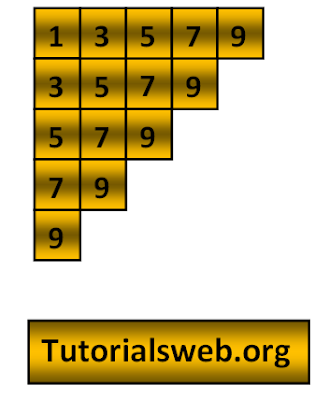## Java Number pattern 16 program using For loop

Write "Java"  program to print below Number Pattern 16:To understand this example, you should have knowledge of the following topics:

·   Java programming operators.

Java Number pattern 16 program using For loop:
`class numberPattern16`
`{`
`public static void main(String[] ags)`
`{`
```    int i,j;
for(i=1;i<=9;i+=2)
{
for(j=i;j<=9;j+=2)
{```
`            System.out.print(j);`
`      }`
`       System.out.println();`
`    }`
`  }`
`}`
Output:
```13579
3579
579
79
9
```
Explanation:
• First two integers "i", and "j" are declared of type int.
• Then in the First for loop “i” value is initialized with a value 9.
• Now “i” value is checked with the condition i <= 9 that is (1 <= 9). So the condition is True.
• Now the loop enters into second for loop and checks the condition j <= 9. Where "j = 1". and the condition is True print the value of "j".
• The value of "j" prints up to condition becomes False.
• Now the value of "i" will be incremented by the condition "i = i+2" and executes the loop.
• when the value of "i"  becomes "11" the program terminates.